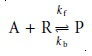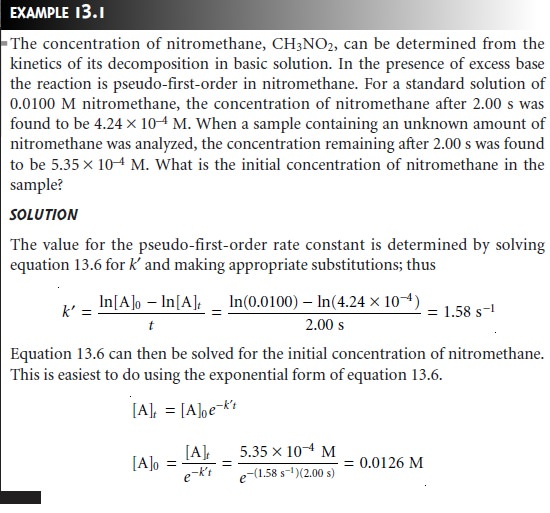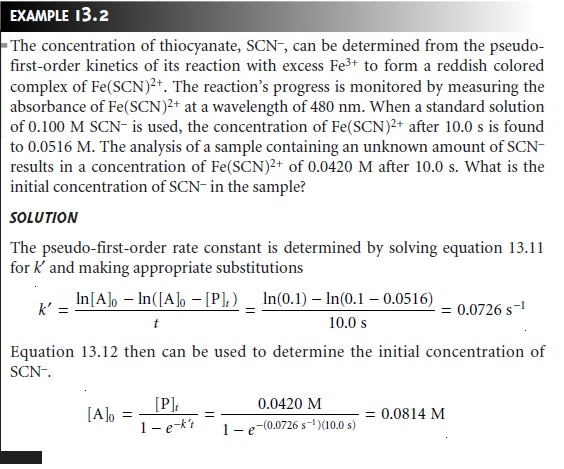Home | | Modern Analytical Chemistry | Methods Based on Chemical Kinetics: Theory and Practice

# Methods Based on Chemical Kinetics: Theory and Practice

Every chemical reaction occurs at a finite rate and, therefore, can potentially serve as the basis for a chemical kinetic method of analysis.

Theory and Practice

Every chemical reaction occurs at a finite rate and, therefore, can potentially serve as the basis for a chemical kinetic method of analysis. To be effective, however, the chemical reaction must meet three conditions. First, the rate of the chemical reac- tion must be fast enough that the analysis can be conducted in a reasonable time, but slow enough that the reaction does not approach its equilibrium position while the reagents are mixing. As a practical limit, reactions reaching equilibrium within 1 s are not easily studied without the aid of specialized equipment allowing for the rapid mixing of reactants.

A second requirement is that the rate law for the chemical reaction must be known for the period in which measurements are made. In addition, the rate law should allow the kinetic parameters of interest, such as rate constants and concen- trations, to be easily estimated. For example, the rate law for a reaction that is first order in the concentration of the analyte, A, is expressed aswhere k is the reaction’s rate constant. As shown in Appendix 5,* the integrated form of this rate law

ln [A]t = ln [A]0 kt

or

[A]t = [A]0ekt     ………..13.2

provides a simple mathematical relationship between the rate constant, the reac- tion’s elapsed time, t, the initial concentration of analyte, [A]0, and the analyte’s concentration at time t, [A]t.

Unfortunately, most reactions of analytical interest do not follow the simple rate laws shown in equations 13.1 and 13.2. Consider, for example, the following re- action between an analyte, A, and a reagent, R, to form a product, Pwhere kf is the rate constant for the forward reaction, and kb is the rate constant for the reverse reaction. If the forward and reverse reactions occur in single steps, then the rate law is

Rate = kf[A][R] kb[P]                        13.3

Although the rate law for the reaction is known, there is no simple integrated form. We can simplify the rate law for the reaction by restricting measurements to the beginning of the reaction when the product’s concentration is negligible. Under these conditions, the second term in equation 13.3 can be ignored; thus

Rate = kf[A][R] t     ………..13.4

The integrated form of the rate law for equation 13.4, however, is still too compli- cated to be analytically useful. We can simplify the kinetics, however, by carefully adjusting the reaction conditions.4 For example, pseudo-first-order kinetics can be achieved by using a large excess of R (i.e. [R]0 >> [A]0), such that its concentration remains essentially constant. Under these conditionsIt may even be possible to adjust conditions such that measurements are made under pseudo-zero-order conditions whereA final requirement for a chemical kinetic method of analysis is that it must be possible to monitor the reaction’s progress by following the change in concentra- tion for one of the reactants or products as a function of time. Which species is used is not important; thus, in a quantitative analysis the rate can be measured by moni- toring the analyte, a reagent reacting with the analyte, or a product. For example, the concentration of phosphate can be determined by monitoring its reaction with Mo(VI) to form 12-molybdophosphoric acid (12-MPA).

H3PO4 + 6Mo(VI) + 9H2O 12-MPA + 9H3O+ t     ………..13.9

We can monitor the progress of this reaction by coupling it to a second reaction in which 12-MPA is reduced to form heteropolyphosphomolybdenum blue, PMB,

12-MPA + nRed → PMB + nOx

where Red is a suitable reducing agent, and Ox is its conjugate form.5,6 The rate of formation of PMB is measured spectrophotometrically and is proportional to the concentration of 12-MPA. The concentration of 12-MPA, in turn, is proportional to the concentration of phosphate. Reaction 13.9 also can be followed spectropho- tometrically by monitoring the formation of 12-MPA.

## Classifying Chemical Kinetic Methods

A useful scheme for classifying chemical ki- netic methods of analysis is shown in Figure 13.3. Methods are divided into two main categories. For those methods identified as direct-computation methods, the concentration of analyte, [A]0, is calculated using the appropriate rate law. Thus, for a first-order reaction in A, equation 13.2 is used to determine [A]0, provided that values for k, t, and [A]t are known. With a curve-fitting method, regression is used to find the best fit between the data (e.g., [A]t as a function of time) and the known mathematical model for the rate law. In this case, kinetic parameters, such as k and [A]0, are adjusted to find the best fit. Both categories are further subdivided into rate methods and integral methods.## Direct-Computation Integral Methods

Integral methods for analyzing kinetic data make use of the integrated form of the rate law. In the one-point fixed-time integral method, the concentration of analyte is determined at a single time. The initial con- centration of analyte, [A]0, is calculated using equation 13.2, 13.6, or 13.8, depend- ing on whether the reaction follows first-order, pseudo-first-order, or pseudo-zero- order kinetics. The rate constant for the reaction is determined in a separate experiment using a standard solution of analyte. Alternatively, the analyte’s initial concentration can be determined using a calibration curve consisting of a plot of [A]t for several standard solutions of known [A]0.In Example 13.1 the initial concentration of analyte is determined by measur- ing the amount of unreacted analyte at a fixed time. Sometimes it is more conven- ient to measure the concentration of a reagent reacting with the analyte or the con- centration of one of the reaction’s products. The one-point fixed-time integral method can still be applied if the stoichiometry is known between the analyte and the species being monitored. For example, if the concentration of the product in the reaction

A+R → P

is monitored, then the concentration of the analyte at time t is

[A]t = [A]0 [P]t     ………..13.10

since the stoichiometry between the analyte and product is 1:1. Substituting equa- tion 13.10 into equation 13.6 gives

ln([A]0 [P]t)= ln [A]0 kt t     ………..13.11

which is simplified by writing in exponential form

[A]0 – [P]t = [A]0ektThe one-point fixed-time integral method has the advantage of simplicity since only a single measurement is needed to determine the analyte’s initial con- centration. As with any method relying on a single determination, however, a one-point fixed-time integral method cannot compensate for constant sources of determinate error. Such corrections can be made by making measurements at two points in time and using the difference between the measurements to determine the analyte’s initial concentration. Constant sources of error affect both measurements equally, thus, the difference between the measurements is independent of these er- rors. For a two-point fixed-time integral method, in which the concentration of an- alyte for a pseudo-first-order reaction is measured at times t1 and t2, we can write

[A]t1 = [A]0ektl t     ………..13.13

[A]t2 = [A]0ekt2

Subtracting the second equation from the first equation and solving for [A]0 givesThe rate constant for the reaction can be calculated from equation 13.14 by measur- ing [A]t1 and [A]t2 for a standard solution of analyte. The analyte’s initial concentra- tion also can be found using a calibration curve consisting of a plot of ([A]t1  [A]t2) versus [A]0.

Fixed-time integral methods are advantageous for systems in which the signal is a linear function of concentration. In this case it is not necessary to determine the concentration of the analyte or product at times t1 or t2, because the relevant con- centration terms can be replaced by the appropriate signal. For example, when a pseudo-first-order reaction is followed spectrophotometrically, when Beer’s law

(Abs)t = εb[A]t

is valid, equations 13.6 and 13.14 can be rewritten as

(Abs)t = [A]0(ektb = c[A]0where (Abs)t is the absorbance at time t, and c is a constant.

An alternative to a fixed-time method is a variable-time method, in which we measure the time required for a reaction to proceed by a fixed amount. In this case the analyte’s initial concentration is determined by the elapsed time, t, with a higher concentration of analyte producing a smaller t. For this reason variable- time integral methods are appropriate when the relationship between the detector’s response and the concentration of analyte is not linear or is unknown. In the one- point variable-time integral method, the time needed to cause a desired change in concentration is measured from the start of the reaction. With the two-point vari- able-time integral method, the time required to effect a change in concentration is measured.

One important application of the variable-time integral method is the quantita- tive analysis of catalysts, which is based on the catalyst’s ability to increase the rate of a reaction. As the initial concentration of catalyst is increased, the time needed to reach the desired extent of reaction decreases. For many catalytic systems the rela tionship between the elapsed time, t, and the initial concentration of analyte iswhere Fcat and Funcat are constants that are functions of the rate constants for the catalyzed and uncatalyzed reactions, and the extent of the reaction during the time span t.## Direct-Computation Rate Methods

Rate methods for analyzing kinetic data are based on the differential form of the rate law. The rate of a reaction at time t, (rate)t, is determined from the slope of a curve showing the change in concentration for a reactant or product as a function of time (Figure 13.5).For a reaction that is first- order, or pseudo-first-order in analyte, the rate at time t is given asSubstituting an equation similar to 13.13 into the preceding equation gives the fol- lowing relationship between the rate at time t and the analyte’s initial concentration.

(rate)t = k[A]0ekt

If the rate is measured at a fixed time, then both k and ekt are constant, and a cali- bration curve of (rate)t versus [A]0 can be used for the quantitative analysis of the analyte.

The use of the initial rate (t = 0) has the advantage that the rate is at its maxi- mum, providing an improvement in sensitivity. Furthermore, the initial rate is measured under pseudo-zero-order conditions, in which the change in concentra- tion with time is effectively linear, making the determination of slope easier. Finally, when using the initial rate, complications due to competing reactions are avoided. One disadvantage of the initial rate method is that there may be insufficient time for a complete mixing of the reactants. This problem is avoided by using a rate mea- sured at an intermediate time (t > 0).## Curve-FittingMethods

In the direct-computation methods discussed earlier, the analyte’s concentration is determined by solving the appropriate rate equa- tion at one or two discrete times. The relationship between the analyte’s concen- tration and the measured response is a function of the rate constant, which must be measured in a separate experiment. This may be accomplished using a single external standard (as in Example 13.2) or with a calibration curve (as in Example 13.4).

In a curve-fitting method the concentration of a reactant or product is moni- tored continuously as a function of time, and a regression analysis is used to fit an appropriate differential or integral rate equation to the data. For example, the initial concentration of analyte for a pseudo-first-order reaction, in which the concentra- tion of a product is followed as a function of time, can be determined by fitting a re- arranged form of equation 13.12

[P]t = [A]0(1 – ekt)

to the kinetic data using both [A]0 and k as adjustable parameters. By using data from more than one or two discrete times, curve-fitting methods are cap- able of producing more reliable results. Although curve-fitting methods are computationally more demanding, the calculations are easily handled by computer.## Miscellaneous Methods

At the beginning of this section we noted that kinetic methods are susceptible to significant errors when experimental variables affecting the reaction’s rate are difficult to control. Many variables, such as temperature, can be controlled with proper instrumentation. Other variables, such as interferents in the sample matrix, are more difficult to control and may lead to significant errors. Although not discussed in this text, direct-computation and curve-fitting methods have been developed that compensate for these sources of error.

## RepresentativeMethod

Although each chemical kinetic method has its own unique considerations, the determination of creatinine in urine based on the ki- netics of its reaction with picrate provides an instructive example of a typical procedure.

Study Material, Lecturing Notes, Assignment, Reference, Wiki description explanation, brief detail
Modern Analytical Chemistry: Kinetic Methods of Analysis : Methods Based on Chemical Kinetics: Theory and Practice |